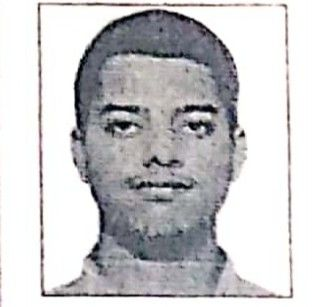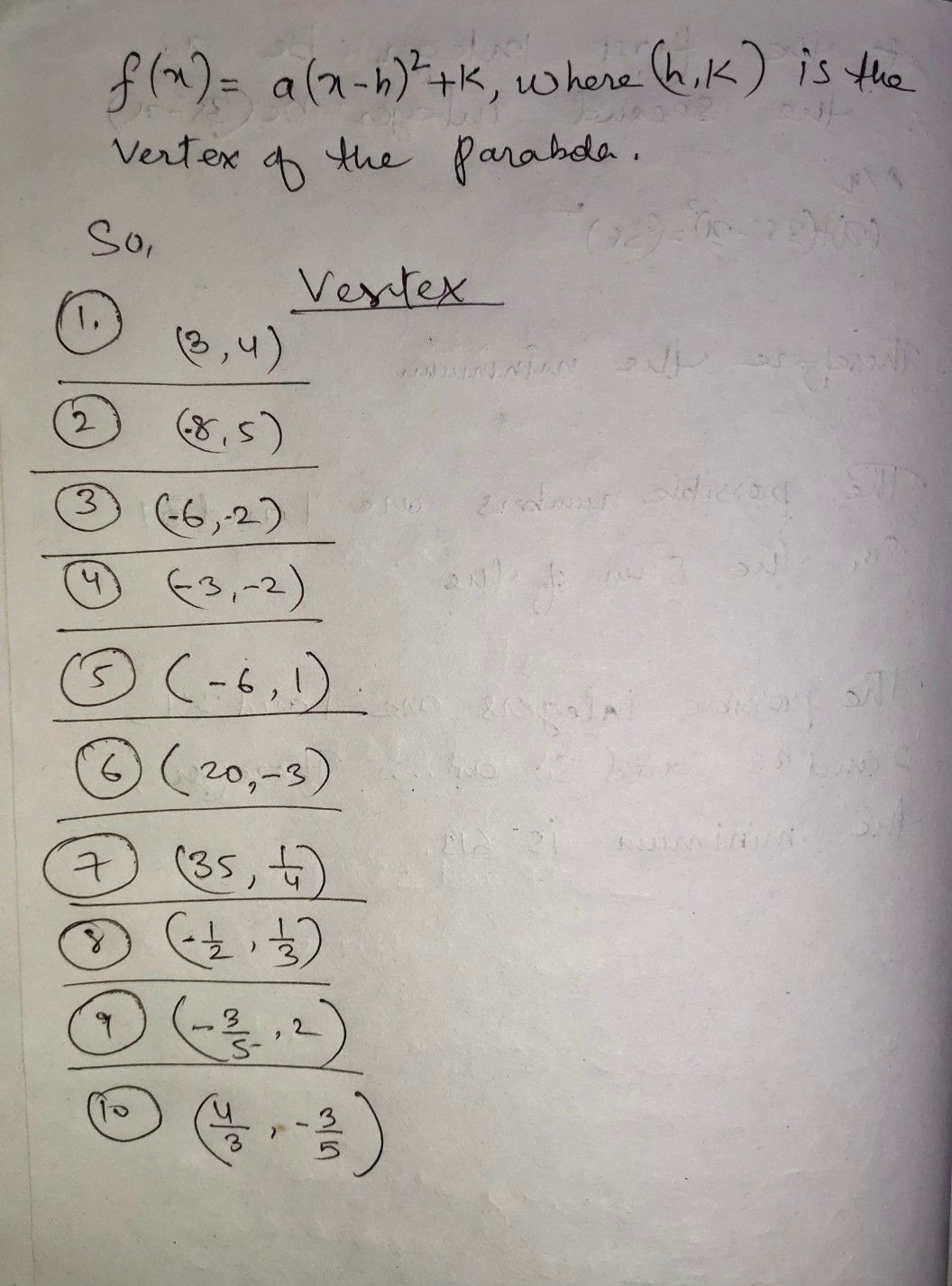Symbol
ProblemACTIVITY $1:1$ What's My Vertex? $Dircciions$ The following are expressed in vertex forms, determ ine the vertex of each item. A. Given Vertex $1.$ $2$ $y=\left(x+3\right)^{2}+4$ $y=\left(x-8\right)^{2}+5$ $3.$ $y=-\left(x+6\right)^{2}-2$ $y=3\left(x-1\right)^{2}-2$ 4. $5.$ $y=-3\left(x+2\right)^{2}+1$ 6. $y=4\left(x+5\right)^{2}$ $+5\right)^{2}-3$ $7.$ $y=-5\left(x-7\right)^{2}+\dfrac {1} {4}$ $8.$ $y=\left(x-\dfrac {1} {2}\right)^{2}+\dfrac {1} {3}$ $9.$ $y=-\left(x+\dfrac {3} {5}\right)^{2}+2$ $10.y=-2\left(x+\dfrac {2} {3}\right)^{2}-\dfrac {3} {5}$
7th-9th grade
Other
Search count: 112
SolutionQanda teacher - amirStudent
legit?